# 3.1 Electrical Conductivity

The standard models for the electrical conductivity have been presented in Section 2.4. These models give very good results for bulk materials, where a single-crystal like behavior or an averaged material behavior can be assumed over the simulation domain. If thin film materials are considered, however, the previously presented description of bulk properties often lacks accuracy especially if the models should cover high-temperature effects. Therefore, additional material models have to be developed, which are able to describe the physics more fundamentally. Such models are intended to be implemented in simulation tools to improve the predictability of material models.

Applying single-crystal material models for bulk-sized materials chunks might can also suit well for semiconductor materials, if the internal microstructure can be neglected. However, if an additional impurity concentration can observed, a significant amount of extra energy barriers are built, as shown in Figure 3.1. Looking at the polycrystalline structure with a broader view the shape of the energy band looks more like Figure 2.12, where the typical ratio of the grain region and the grain boundaries is depicted. Here, the grains are separated by an energetic barrier consisting mostly of mismatched atoms that are located with a certain offset to the optimal lattice sites of an ideal crystal. Due to the diffusion and the segregation tendency of the impurities in the semiconductor materials, these impurity atoms move towards the interfaces of the grains and build structures as shown in Figure 3.1 at grain boundaries and at the surfaces.

To account for this type of behavior, the polycrystalline structure has been considered for contacts in transistors where additional barriers occur besides the work-function difference [177,178]. This model can be applied to other material parts where polycrystalline Si appears, for instance in contacts . Consequently, a model has been developed by MANDURAH and others, which accounts for these energetic barriers so as to derive a macroscopic model that can be calibrated if the necessary information is provided by measurements (cf. (3.3) and [179,180,181,177,178]). Based on this model NATHAN and BALTES propose in  to use the assumptions of MANDURAH from [179,180] and extend the grain boundary model by including an average-sized grain to obtain a more reasonable conductivity model.

Applications for such models include solar cells, polycrystalline diodes for temperature measurements, fuses, and sensors in general. The advantage of this model is to predict the behavior of the polycrystalline semiconductor materials in regions where single crystal growth cannot be provided. In this case, the electrical behavior is different from devices built from single-crystal materials. For instance, the electrical resistivity highly depends on the temperature and thus also on the reverse current of a diode made of polycrystalline Si.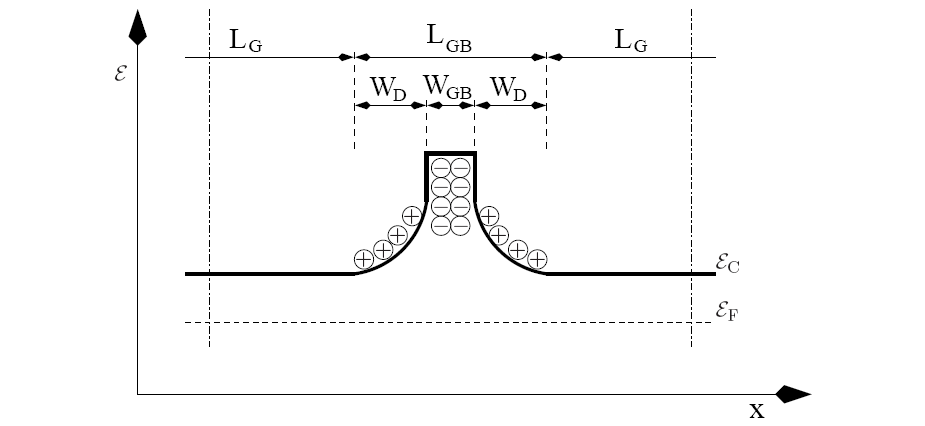When comparing the standard model with measurements of polycrystalline materials, the original polygonal model with a reference value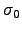and thermal correction term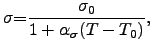(3.1)

yields acceptable results for a very limited temperature range. Here,denotes the electrical conductivity at the reference temperature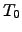,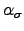is the material-dependent temperature coefficient of first order for the electrical conductivity,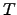is the absolute temperature. Depending on the shape of the real conductivity, the temperature coefficienthas a certain range of validity. If wider ranges are considered, a second order temperature coefficient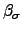can be introduced by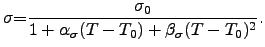(3.2)

Regardless of the temperature bias point (reference temperature) assumed, specific different values for,, andare required. They can be obtained by model calibrationa with measuremnts or data listed in literature.

Yet this expansion has its limits. For applications where this second-order temperature coefficient model is not sufficiently accurate, the microscopic structure of the material has to be included into the conductivity model. Especially if material compounds are considered, e.g. polycrystalline silicon with high doping for a better conduction, or silicided metals (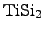,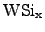), the thermal impact on the conductivity can no longer be described by these polynomial functions. With inclusion of the microstructure into the electrical models, the accuracy of the models can be increased. As proposed in , a conductivity model which accounts for grain boundaries [179,180,177] is combined with the drift diffusion model for the plain grains.

The grain boundary model for the electrical conductivity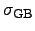considers a ballistic transport over the barriers which can be described by the doping concentration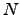, the interface trap density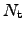, and by the temperature. The other parameters are more or less constants for a certain process technology. Following [179,180], the model for the grain boundary conductivity reads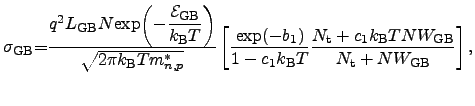(3.3)

where the coefficients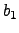and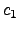are defined as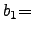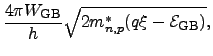(3.4)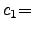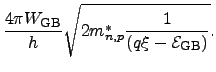(3.5)

The first term in (3.3) determines the base value of the conductivity due to the ballistic transport over the energy barrier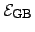. However, this base value is corrected by two additional factors, where the first correction factor is to due the temperature dependence of the width of the depletion zone close to the grain boundary and the second correction is due to the temperature dependence of the trap density in the interface region between the grain boundary and the grain. In these equations, the energy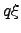is the grain boundary energy level defined relative to the conduction band . The effective mass for electrons and holes are denoted as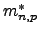,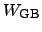is the grain barrier width,the overall length of the grain boundary including the depletion zone, and the width of the depletion zone is defined as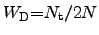according to .

To determine the global conductivity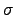of a polycrystalline material, NATHAN and BALTES  used the MATTHIESSEN rule to combine the electrical conductivities of the grain regionobtained from (2.14) and (2.15) and the grain boundary regionas(3.6)

where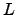represents the overall length of a unit cell,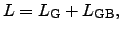(3.7)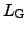denotes the average length of a grain andthe average width of a grain boundary. However, to consider also the third dimension, (3.6) can be extended by using the same assumptions in three dimensions. Hence, a cube with an edge length of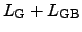is considered, which consists of a cubic-shaped grain with the edge lengthand the remaining part represents the contribution of the barrier. Considering a cross sectional cut perpendicular to the current density, the sheet conductivity for electrons and holes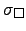can be expressed by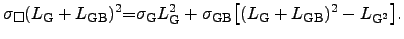(3.8)

Thus, the overall conductivitywith the corrected terms is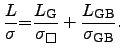(3.9)

However, the use of this model has also its limitations. The model has shown to be rather sensitive to changes of the parameters. For instance the average grain size has a significant impact on the value of the conductivity of this model. Due to different process steps and fabrication formulas, the parameters for this model can change significantly, which requires a separate parameter extraction for each particular setting of the machines used in the fabrication of the microelectronic device. Nevertheless, the model provides a better insight in to the microstructure of polycrystalline materials using a meso scale model.

Stefan Holzer 2007-11-19